Simple Nested Loops Join Block Nested Loops Join

• Slides: 24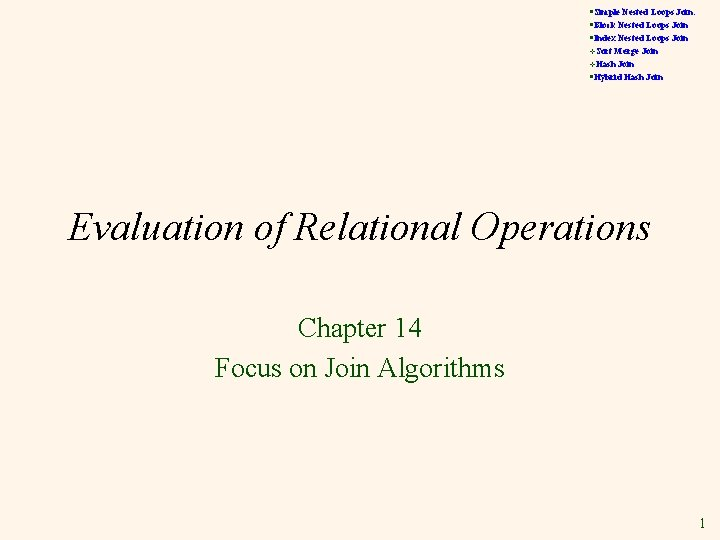§Simple Nested Loops Join: §Block Nested Loops Join §Index Nested Loops Join v. Sort Merge Join §Hybrid Hash Join v. Hash Evaluation of Relational Operations Chapter 14 Focus on Join Algorithms 1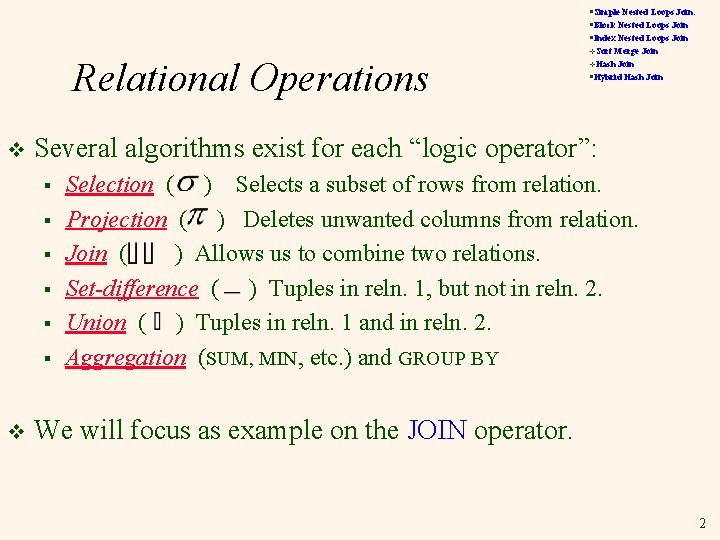§Simple Nested Loops Join: §Block Nested Loops Join §Index Nested Loops Join Relational Operations v Merge Join §Hybrid Hash Join v. Hash Several algorithms exist for each “logic operator”: § § § v v. Sort Selection ( ) Selects a subset of rows from relation. Projection ( ) Deletes unwanted columns from relation. Join ( ) Allows us to combine two relations. Set-difference ( ) Tuples in reln. 1, but not in reln. 2. Union ( ) Tuples in reln. 1 and in reln. 2. Aggregation (SUM, MIN, etc. ) and GROUP BY We will focus as example on the JOIN operator. 2§Simple Nested Loops Join: §Block Nested Loops Join §Index Nested Loops Join Schema for Examples v. Sort Merge Join §Hybrid Hash Join v. Hash Sailors (sid: integer, sname: string, rating: integer, age: real) Reserves (sid: integer, bid: integer, day: dates, rname: string) Similar to old schema; rname added for variations. v Reserves: v § v Each tuple is 40 bytes long, 100 tuples per page, 1000 pages. Sailors: § Each tuple is 50 bytes long, 80 tuples per page, 500 pages. 3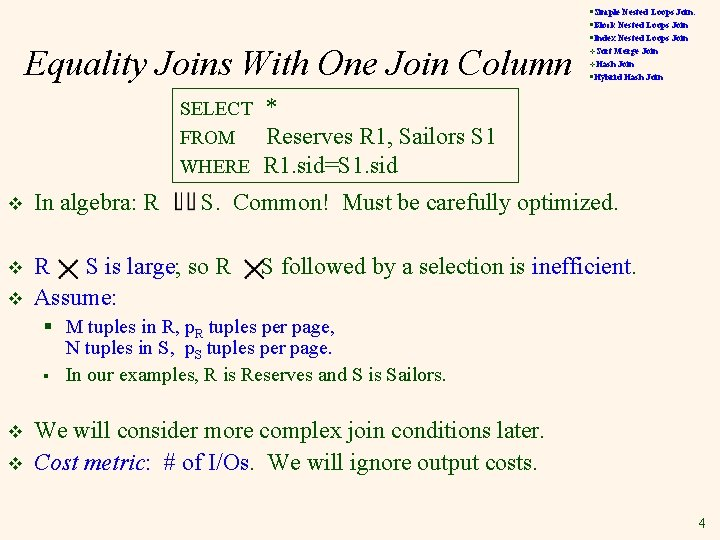Equality Joins With One Join Column SELECT FROM WHERE v In algebra: R v R S is large; so R Assume: v §Simple Nested Loops Join: §Block Nested Loops Join §Index Nested Loops Join v. Sort Merge Join §Hybrid Hash Join v. Hash * Reserves R 1, Sailors S 1 R 1. sid=S 1. sid S. Common! Must be carefully optimized. S followed by a selection is inefficient. § M tuples in R, p. R tuples per page, N tuples in S, p. S tuples per page. § In our examples, R is Reserves and S is Sailors. v v We will consider more complex join conditions later. Cost metric: # of I/Os. We will ignore output costs. 4§Simple Nested Loops Join: §Block Nested Loops Join §Index Nested Loops Join Typical Choices v v. Sort Merge Join §Hybrid Hash Join v. Hash Nested Loops Join § Simple Nested Loops Join: Tuple-oriented, Page-oriented § Block Nested Loops Join § Index Nested Loops Join Sort Merge Join v Hash Join v § Hybrid Hash Join 5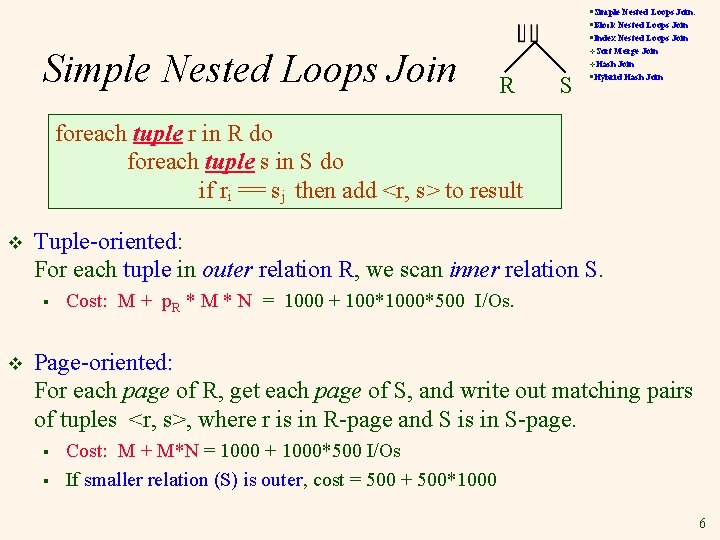§Simple Nested Loops Join: §Block Nested Loops Join §Index Nested Loops Join Simple Nested Loops Join v. Sort Merge Join §Hybrid Hash Join v. Hash R S foreach tuple r in R do foreach tuple s in S do if ri == sj then add <r, s> to result v Tuple-oriented: For each tuple in outer relation R, we scan inner relation S. § v Cost: M + p. R * M * N = 1000 + 100*1000*500 I/Os. Page-oriented: For each page of R, get each page of S, and write out matching pairs of tuples <r, s>, where r is in R-page and S is in S-page. § § Cost: M + M*N = 1000 + 1000*500 I/Os If smaller relation (S) is outer, cost = 500 + 500*1000 6§Simple Nested Loops Join: §Block Nested Loops Join §Index Nested Loops Join Block Nested Loops Join v v. Sort R S One page as input buffer for scanning inner S, one page as the output buffer, remaining pages to hold ``block’’ of outer R. § § § Merge Join §Hybrid Hash Join v. Hash For each matching tuple r in R-block, s in S-page, add <r, s> to result. Then read next R-block, scan S again. Etc. Find matching tuple? Use in-memory hashing. R&S Hash table for block of R (k < B-1 pages) Join Result . . Input buffer for S Output buffer 7Examples of Block Nested Loops v § § #outer blocks = Cost of scanning R is 1000 I/Os; a total of 10 blocks. Per block of R, we scan Sailors (S); 10*500 I/Os. E. g. , If a block is 90 pages of R, we would scan S 12 times. With 100 -page block of Sailors as outer: § § v Merge Join §Hybrid Hash Join v. Hash With Reserves (R) as outer, and 100 pages of R as block: § v v. Sort Cost: Scan of outer + #outer blocks * scan of inner § v §Simple Nested Loops Join: §Block Nested Loops Join §Index Nested Loops Join Cost of scanning S is 500 I/Os; a total of 5 blocks. Per block of S, we scan Reserves; 5*1000 I/Os. Optimizations? § With sequential reads considered, analysis changes: may be best to divide buffers evenly between R and S. § Double buffering would also be suitable. 8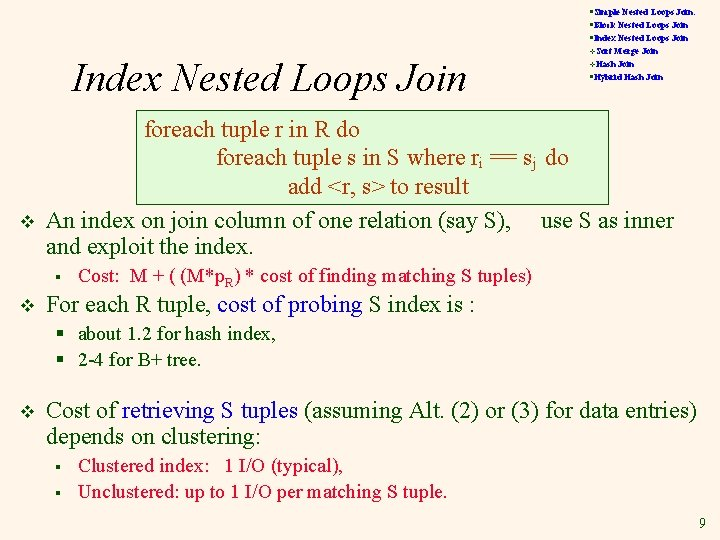§Simple Nested Loops Join: §Block Nested Loops Join §Index Nested Loops Join v Merge Join §Hybrid Hash Join v. Hash foreach tuple r in R do foreach tuple s in S where ri == sj do add <r, s> to result An index on join column of one relation (say S), use S as inner and exploit the index. § v v. Sort Cost: M + ( (M*p. R) * cost of finding matching S tuples) For each R tuple, cost of probing S index is : § about 1. 2 for hash index, § 2 -4 for B+ tree. v Cost of retrieving S tuples (assuming Alt. (2) or (3) for data entries) depends on clustering: § § Clustered index: 1 I/O (typical), Unclustered: up to 1 I/O per matching S tuple. 9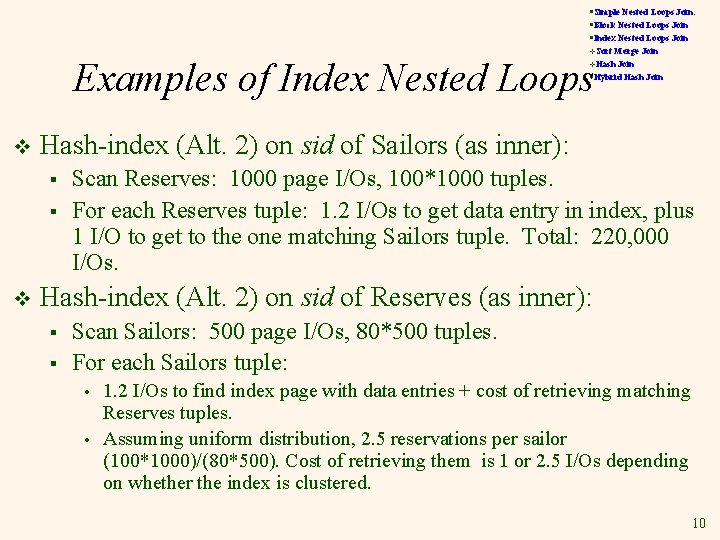§Simple Nested Loops Join: §Block Nested Loops Join §Index Nested Loops Join v. Sort Merge Join §Hybrid Hash Join Examples of Index Nested Loops v. Hash v Hash-index (Alt. 2) on sid of Sailors (as inner): § § v Scan Reserves: 1000 page I/Os, 100*1000 tuples. For each Reserves tuple: 1. 2 I/Os to get data entry in index, plus 1 I/O to get to the one matching Sailors tuple. Total: 220, 000 I/Os. Hash-index (Alt. 2) on sid of Reserves (as inner): § § Scan Sailors: 500 page I/Os, 80*500 tuples. For each Sailors tuple: • • 1. 2 I/Os to find index page with data entries + cost of retrieving matching Reserves tuples. Assuming uniform distribution, 2. 5 reservations per sailor (100*1000)/(80*500). Cost of retrieving them is 1 or 2. 5 I/Os depending on whether the index is clustered. 10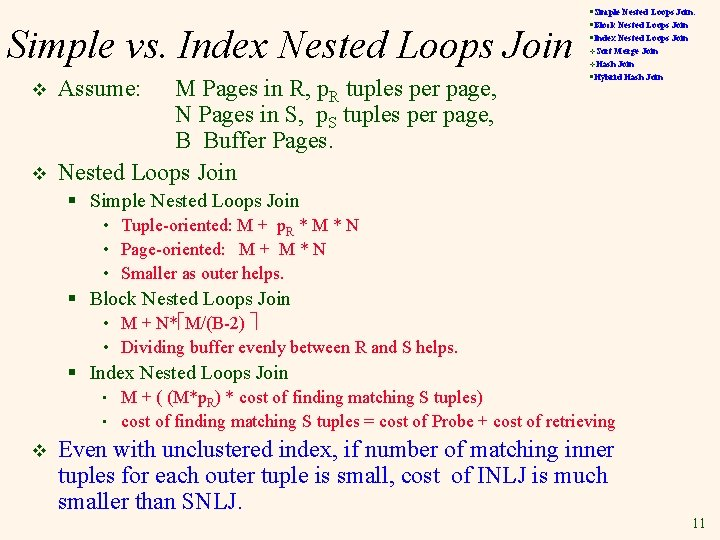Simple vs. Index Nested Loops Join v v Assume: M Pages in R, p. R tuples per page, N Pages in S, p. S tuples per page, B Buffer Pages. Nested Loops Join §Simple Nested Loops Join: §Block Nested Loops Join §Index Nested Loops Join v. Sort Merge Join §Hybrid Hash Join v. Hash § Simple Nested Loops Join • Tuple-oriented: M + p. R * M * N • Page-oriented: M + M * N • Smaller as outer helps. § Block Nested Loops Join • M + N* M/(B-2) • Dividing buffer evenly between R and S helps. § Index Nested Loops Join • • v M + ( (M*p. R) * cost of finding matching S tuples) cost of finding matching S tuples = cost of Probe + cost of retrieving Even with unclustered index, if number of matching inner tuples for each outer tuple is small, cost of INLJ is much smaller than SNLJ. 11Join: Sort-Merge (R i=j S) §Simple Nested Loops Join: §Block Nested Loops Join §Index Nested Loops Join v. Sort Merge Join §Hybrid Hash Join v. Hash (1). Sort R and S on the join column. (2). Scan R and S to do a ``merge’’ on join col. (3). Output result tuples. § Merge on Join Column: • • At this point, all R tuples with same value in Ri (current R group) and all S tuples with same value in Sj (current S group) match; So output <r, s> for all pairs of such tuples. • Then resume scanning R and S (as above) • v Advance scan of R until current R-tuple >= current S tuple, then advance scan of S until current S-tuple >= current R tuple; do this until current R tuple = current S tuple. R is scanned once; each S group is scanned once per matching R tuple. (Multiple scans of an S group are likely to find needed pages in buffer. ) 12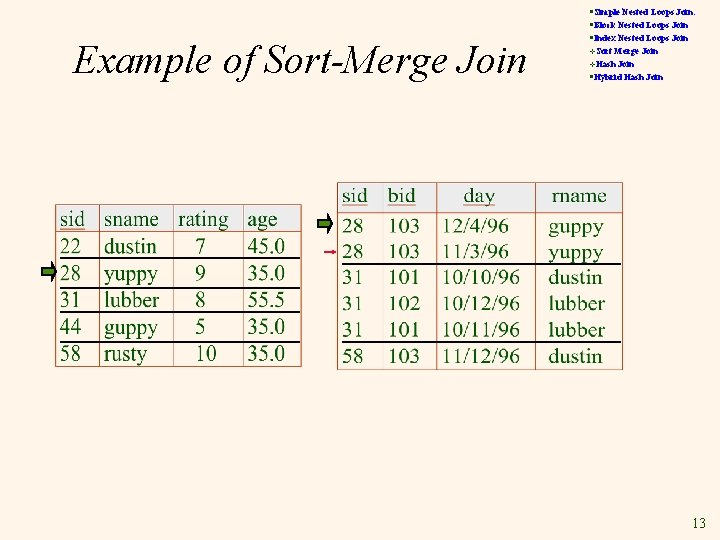Example of Sort-Merge Join §Simple Nested Loops Join: §Block Nested Loops Join §Index Nested Loops Join v. Sort Merge Join §Hybrid Hash Join v. Hash 13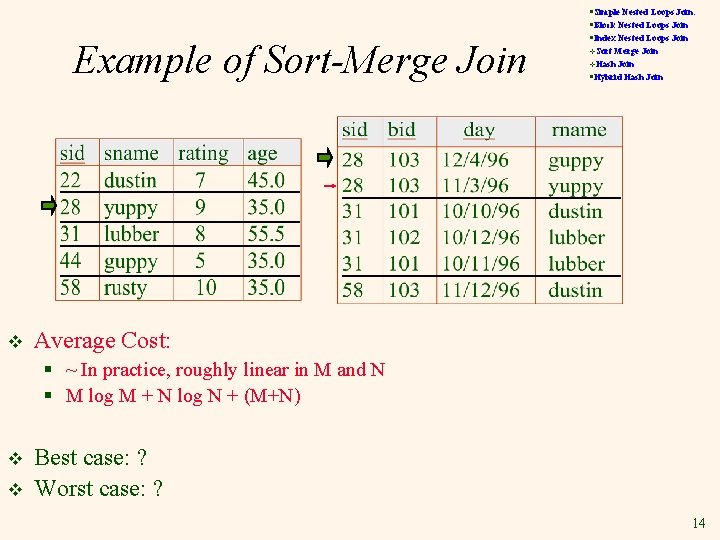Example of Sort-Merge Join v §Simple Nested Loops Join: §Block Nested Loops Join §Index Nested Loops Join v. Sort Merge Join §Hybrid Hash Join v. Hash Average Cost: § ~ In practice, roughly linear in M and N § M log M + N log N + (M+N) v v Best case: ? Worst case: ? 14§Simple Nested Loops Join: §Block Nested Loops Join §Index Nested Loops Join Problem of Sort-Merge Join v § The cost of scanning, M+N Could be M*N R v v Merge Join §Hybrid Hash Join v. Hash Average Cost: M log M + N log N + (M+N) § v v. Sort S Many pages in R in same partition. ( Worst, All of them). The pages for this partition in S don’t fit into RAM. Re-scan S is needed. Multiple scan S is expensive! Worst Case: M*N Can guarantee M+N if key-FK join, or no duplicates. 15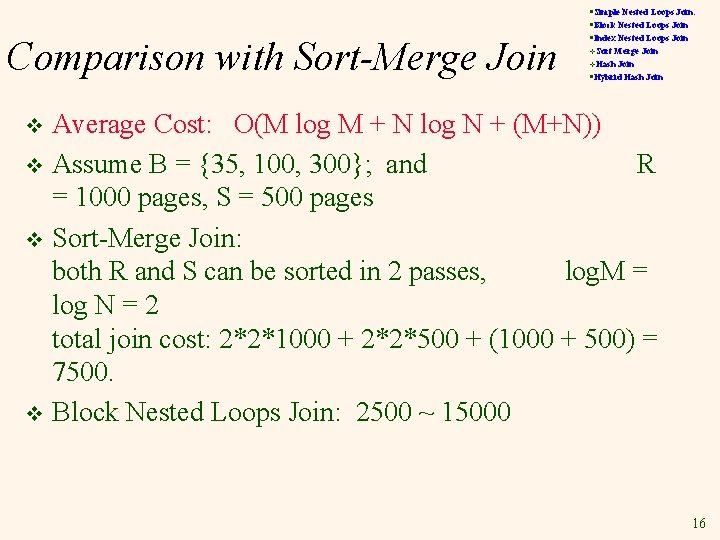Comparison with Sort-Merge Join §Simple Nested Loops Join: §Block Nested Loops Join §Index Nested Loops Join v. Sort Merge Join §Hybrid Hash Join v. Hash Average Cost: O(M log M + N log N + (M+N)) v Assume B = {35, 100, 300}; and R = 1000 pages, S = 500 pages v Sort-Merge Join: both R and S can be sorted in 2 passes, log. M = log N = 2 total join cost: 2*2*1000 + 2*2*500 + (1000 + 500) = 7500. v Block Nested Loops Join: 2500 ~ 15000 v 16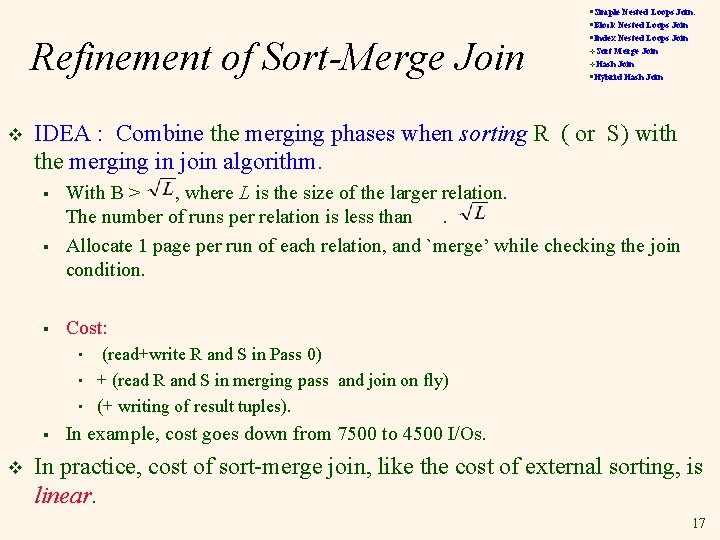Refinement of Sort-Merge Join v v. Sort Merge Join §Hybrid Hash Join v. Hash IDEA : Combine the merging phases when sorting R ( or S) with the merging in join algorithm. § § § With B > , where L is the size of the larger relation. The number of runs per relation is less than. Allocate 1 page per run of each relation, and `merge’ while checking the join condition. Cost: • • • § v §Simple Nested Loops Join: §Block Nested Loops Join §Index Nested Loops Join (read+write R and S in Pass 0) + (read R and S in merging pass and join on fly) (+ writing of result tuples). In example, cost goes down from 7500 to 4500 I/Os. In practice, cost of sort-merge join, like the cost of external sorting, is linear. 17Hash-Join Partition both relations using same hash fn h: R tuples in partition i will only match S tuples in partition i. Original Relation OUTPUT 1 1 2 INPUT 2 hash function . . . h B-1 Disk B main memory buffers Partitions of R & S v Partitions Read in a partition of R, hash it using h 2 (<> h!). Scan matching partition of S, search for matches. Disk Join Result hash fn Hash table for partition Ri (k < B-1 pages) h 2 Input buffer for Si Disk Output buffer B main memory buffers Disk§Simple Nested Loops Join: §Block Nested Loops Join §Index Nested Loops Join Cost of Hash-Join v v. Sort Merge Join §Hybrid Hash Join v. Hash In partitioning phase, read+write both relations: § 2(M+N). v In matching phase, read both relations: § M+N I/Os. v Total : 3(M+N) v E. g. , total of 4500 I/Os in our running example. 19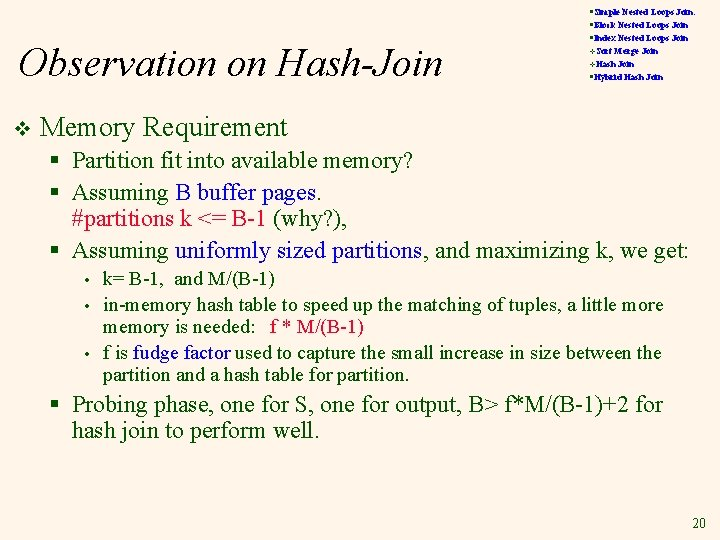Observation on Hash-Join v §Simple Nested Loops Join: §Block Nested Loops Join §Index Nested Loops Join v. Sort Merge Join §Hybrid Hash Join v. Hash Memory Requirement § Partition fit into available memory? § Assuming B buffer pages. #partitions k <= B-1 (why? ), § Assuming uniformly sized partitions, and maximizing k, we get: • • • k= B-1, and M/(B-1) in-memory hash table to speed up the matching of tuples, a little more memory is needed: f * M/(B-1) f is fudge factor used to capture the small increase in size between the partition and a hash table for partition. § Probing phase, one for S, one for output, B> f*M/(B-1)+2 for hash join to perform well. 20§Simple Nested Loops Join: §Block Nested Loops Join §Index Nested Loops Join Observation on Hash Join v v. Sort Merge Join §Hybrid Hash Join v. Hash Overflow § If the hash function does not partition uniformly, one or more R partitions may not fit in memory. § Significantly degrade the performance. § Can apply hash-join technique recursively to do the join of this overflow R-partition with corresponding Spartition. 21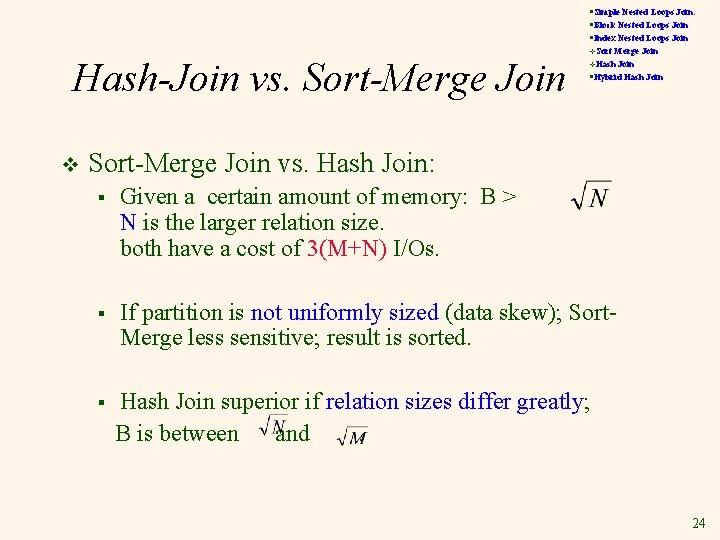§Simple Nested Loops Join: §Block Nested Loops Join §Index Nested Loops Join Hash-Join vs. Sort-Merge Join v v. Sort Merge Join §Hybrid Hash Join v. Hash Sort-Merge Join vs. Hash Join: § Given a certain amount of memory: B > N is the larger relation size. both have a cost of 3(M+N) I/Os. § If partition is not uniformly sized (data skew); Sort. Merge less sensitive; result is sorted. § Hash Join superior if relation sizes differ greatly; B is between and. 24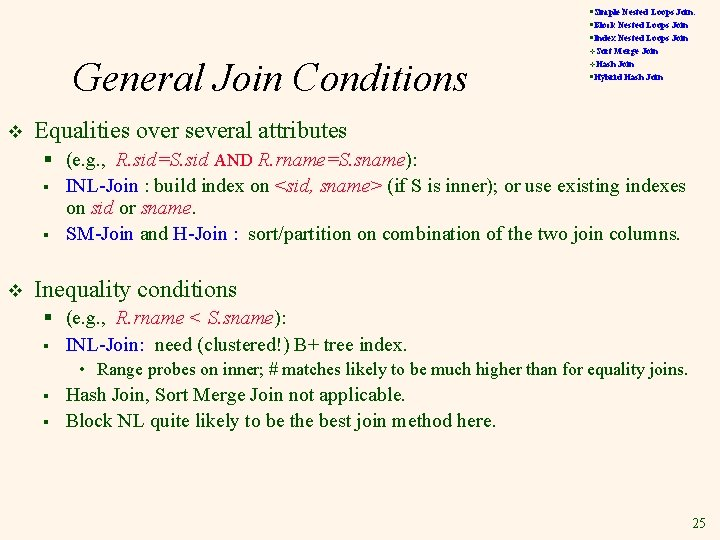§Simple Nested Loops Join: §Block Nested Loops Join §Index Nested Loops Join General Join Conditions v v. Sort Merge Join §Hybrid Hash Join v. Hash Equalities over several attributes § (e. g. , R. sid=S. sid AND R. rname=S. sname): § INL-Join : build index on <sid, sname> (if S is inner); or use existing indexes on sid or sname. § SM-Join and H-Join : sort/partition on combination of the two join columns. v Inequality conditions § (e. g. , R. rname < S. sname): § INL-Join: need (clustered!) B+ tree index. • Range probes on inner; # matches likely to be much higher than for equality joins. § § Hash Join, Sort Merge Join not applicable. Block NL quite likely to be the best join method here. 25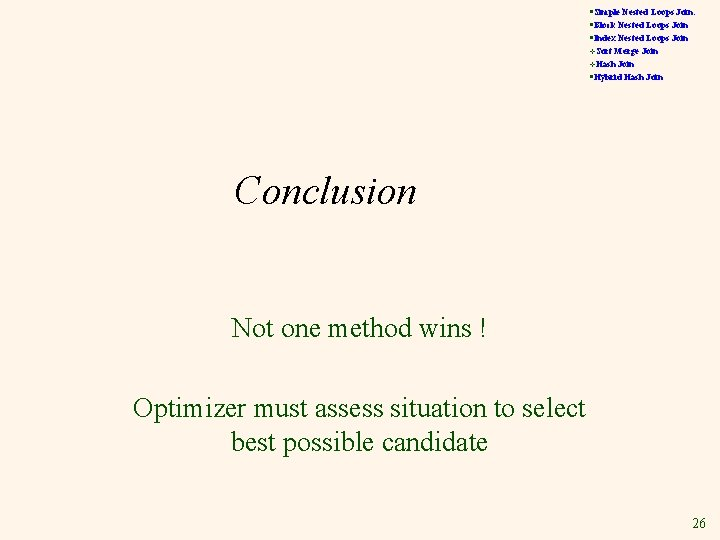§Simple Nested Loops Join: §Block Nested Loops Join §Index Nested Loops Join v. Sort Merge Join §Hybrid Hash Join v. Hash Conclusion Not one method wins ! Optimizer must assess situation to select best possible candidate 26